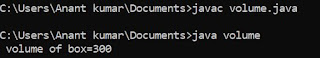## Tuesday, 24 July 2018

### What are Armstrong number ?

An armstrong number is a number which is equal to the sum of digit rise to the power of total number of digit in the number.

Example :: 3 , 370 , 153

### Program to check armstrong number ::

#include <stdio.h>
#include <math.h>
void main ()
{
int n , add = 0 , rem = 0 , temp , cube , pow ;
clrscr () ;
printf ('\n  Enter a number:") ;
scanf ("%d", &n) ;
temp = n ;
while ( n != 0 )
{
rem = n % 10 ;
cube = pow ( rem , 3 ) ;
n = n / 10 ;
}
if ( add == temp )
printf ("\n Number is armstrong:") ;
else
printf ("\n Number is not armstrong:") ;
getch ()
}

OUTPUT :: (A)  Enter a number :-
Enter number is 153
Number is armstrong

(B) Enter a number :-
Enter number is 29
Number is not armstrong

### C program of armstrong using concept of FUNCTION ::

When a program grow in size and complexity then we break that program into small module . These module are known as the function . Function increases the understandably of a program logic using function module independent logic can be defined .

Function provides the concept of modularity in a program which is best method to divide a complex program .
#include <stdio.h>
int root ( int , int ) ;
void main ()
{
int n , add = 0 , temp , rem , word = 0 ;
clrscr () ;
printf ("\n Enter a number:") ;
scanf ("%d", &n) ;
temp = n ;
while ( temp != 0 )
{
word++ :
temp = temp / 10 ;
}
temp = n ;
while ( temp != 0 )
{
rem = temp % 10 ;
add = add + root ( remainder , digit ) ;
temp = temp / 10 ;
}
if ( n == add )
printf ("\n armstrong number:") ;
else
printf ("\n not armstrong number:") ;
}
int root ( int n , int r )
{
int c , p = 1 ;
for ( c = 1 : c <= r : c++ )
p = p * n ;
return p ;
}
getch () ;
}